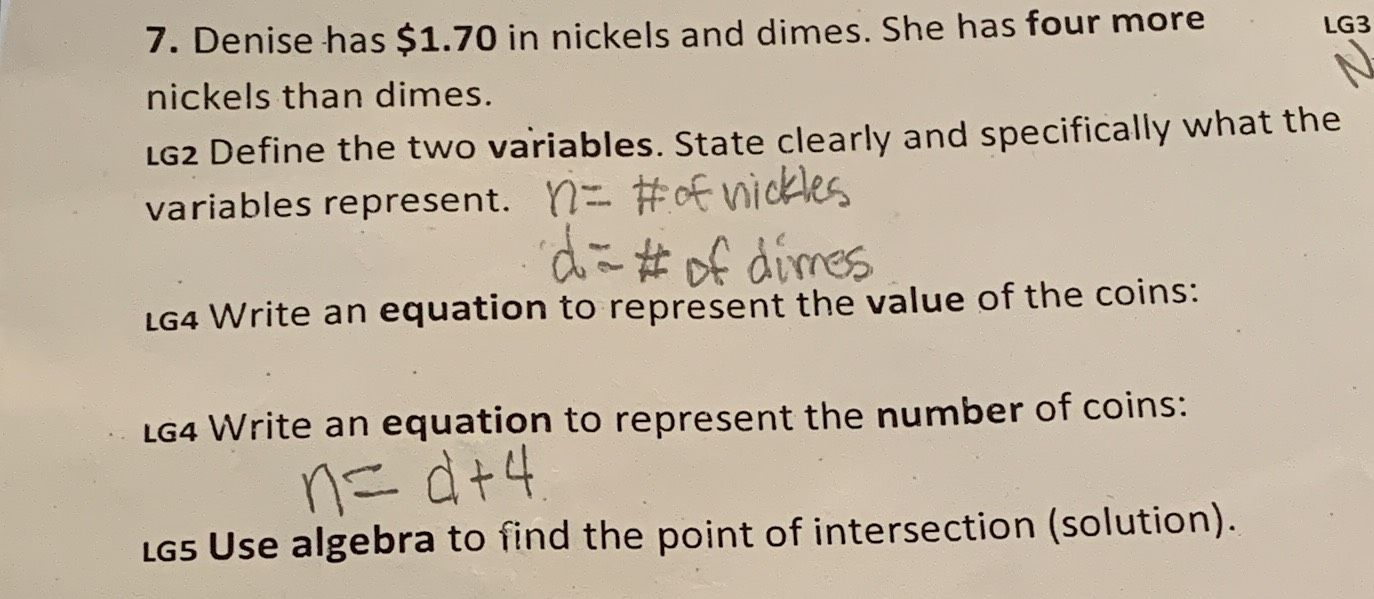### Still have math questions?

Algebra
QuestionDenise has $$\ 1.70$$ in nickels and dimes. She has four more nickels than dimes.

LG2 Define the two variables. State clearly and specifically what the variables represent.

LG4 Write an equation to represent the value of the coins:

LG4 Write an equation to represent the number of coins:

LG5 Use algebra to find the point of intersection (solution).

$$0.05n+ 0.1(n- 4)= 1.7\\n= 14$$